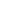# Free CBSE Class 5 Maths Worksheets

Download free printable Maths Worksheets to practice. With thousands of questions available, you can generate as many Maths Worksheets as you want.

## Sample CBSE Class 5 Maths Worksheet Questions

1.

3=2=5

1. True 2. False
2.

Find the perimeter of the figure?3.

Find the perimeter of the figure?4.

Find the perimeter of the figure.5.

Find the perimeter of each of the following.6.

Find the perimeter of the figure.7.

Find the perimeter of each of the given figure8.

Match the following9.

Complete the following table for rectangles.10.

Complete the following table for squares.## Find more Maths Worksheets

Worksheets by UrbanPro

Our worksheets are designed to help students explore various topics, practice skills and enrich their subject knowledge, to improve their academic performance. Designed by Experts who have extensive experience and expertise in teaching a subject, these worksheets will improve your child's problem-solving skills and subject knowledge in a fun and interactive manner.
Check out our free customized worksheets across school boards, grades, subjects and levels of subject knowledge. You can download, print and share these worksheets with anyone, anywhere, anytime!

Get a custom worksheet to practice!

Select your topic & see the magic.

subjectSelect Chapter(s)

Chapters & Subtopics